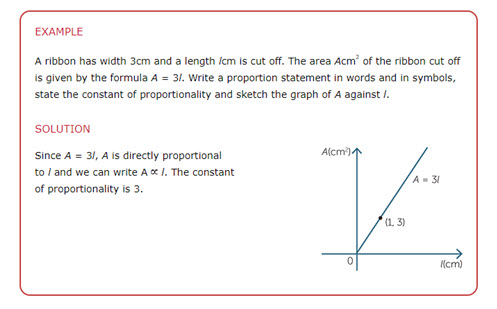Go to website

# Proportion

This module from the AMSI 'The Improving Mathematics Education in Schools (TIMES) project' is for teachers who wish to consolidate their content knowledge on the topic of proportion and how to use and illustrate proportional relationships. There are sections that provide history and context for the topic, as well as exercises and examples of how to present and explain the concepts to students.

Year level(s) Year 8, Year 9
Purpose Content knowledge
Keywords direct proportion, relationship, graph, explicit teaching

## Curriculum alignment

Strand and focus Number
Topics Proportion, rates and ratios
AC: Mathematics (V9.0) content descriptions
AC9M8A02
Graph linear relations on the Cartesian plane using digital tools where appropriate; solve linear equations and one-variable inequalities using graphical and algebraic techniques; verify solutions by substitution

AC9M8N05

Use mathematical modelling to solve practical problems involving rational numbers and percentages, including financial contexts; formulate problems, choosing efficient calculation strategies and using digital tools where appropriate; interpret and communicate solutions in terms of the situation, reviewing the appropriateness of the model

AC9M9A04
Identify and graph quadratic functions, solve quadratic equations graphically and numerically, and solve monic quadratic equations with integer roots algebraically, using graphing software and digital tools as appropriate

AC9M9M05
Use mathematical modelling to solve practical problems involving direct proportion, rates, ratio and scale, including financial contexts; formulate the problems and interpret solutions in terms of the situation; evaluate the model and report methods and findings

Numeracy progression Number patterns and algebraic thinking (P7, P8)
Proportional thinking (P6, P7)
Understanding geometric properties (P7)
Understanding money (P8, P9)